# How do you write big brackets in LaTeX?

If the size and shape of the expression are large, the size of the bracket should also be large. And big brackets will be needed in the latex document to represent multiple line expressions.

\documentclass{article}
\begin{document}
$(a), [b], \{c\}, \langle d \rangle$
$(\frac{X_i}{K_1})$
$\sum_{i=1}^n [\frac{x}{y_n}]$
$\{ \frac{1}{2}, \frac{1}{3}, \frac{1}{4}, \dots \}$
$\langle \frac{2}{3}, \frac{3}{4} \rangle$
\end{document}

Output :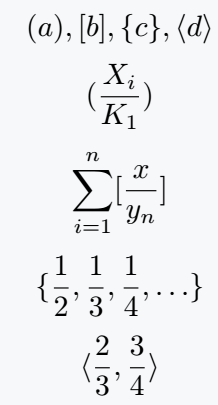Notice the output above where your problem is described. And below is the use of \left and \right command to solve your problem.

\documentclass{article}
\begin{document}
$\left(\frac{X_i}{K_1}\right)$
$\sum_{i=1}^n \left[\frac{x}{y_n}\right]$
$\left\{ \frac{1}{2}, \frac{1}{3}, \frac{1}{4}, \dots \right\}$
$\left\langle \frac{2}{3}, \frac{3}{4} \right\rangle$
\end{document}

Output :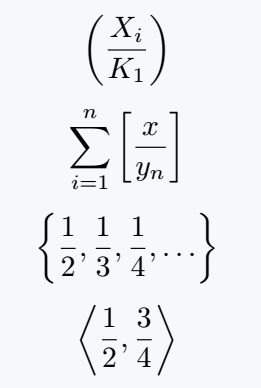For Big Brackets, there is no need to write large syntax in a latex document more than once. Instead, you can create small macros.

\documentclass{article}
\newcommand\Pbracket{\left(#1\right)}
\newcommand\Sbracket{\left[#1\right]}
\newcommand\Cbracket{\left\{#1\right\}}
\newcommand\Lbracket{\left\langle#1\right\rangle}
\begin{document}
$\Pbracket{\frac{X_i}{K_1}}$
$\sum_{i=1}^n \Sbracket{\frac{x}{y_n}}$
$\Cbracket{\frac{1}{2}, \frac{1}{3}, \frac{1}{4}, \dots}$
$\Lbracket{\frac{1}{2}, \frac{3}{4}}$
\end{document}

Output :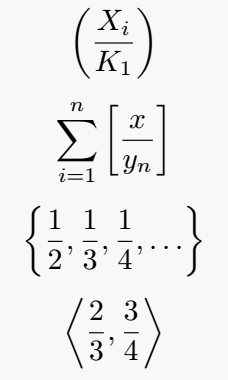## Various types of big commands in LaTeX

There are four types of big commands that do not depend on the expression. Size of bracket is not dynamically adjustable with the size of expression.

\documentclass{article}
\begin{document}
$\big(\frac{a_1}{k}\big), \Big(\frac{a_2}{k}\Big), \bigg(\frac{a_3}{k}\bigg), \Bigg(\frac{a_4}{k}\Bigg)$
$\big[\frac{b_1}{k}\big], \Big[\frac{b_2}{k}\Big], \bigg[\frac{b_3}{k}\bigg], \Bigg[\frac{b_4}{k}\Bigg]$
$\big\{\frac{c_1}{k}\big\}, \Big\{\frac{c_2}{k}\Big\}, \bigg\{\frac{c_3}{k}\bigg\}, \Bigg\{\frac{c_4}{k}\Bigg\}$
$\big\langle\frac{d_1}{k}\big\rangle, \Big\langle\frac{d_2}{k}\Big\rangle, \bigg\langle\frac{d_3}{k}\bigg\rangle, \Bigg\langle\frac{d_4}{k}\Bigg\rangle$
\end{document}

Output :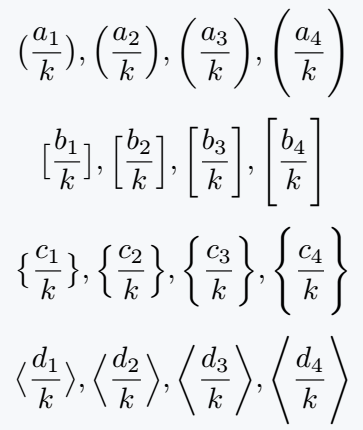## Use Big Brackets in multiple lines equation

No matter what environment you use, LaTeX has some rules for using big brackets with multiline equations.

\left and \right will be used simultaneously in each line. So, if there is no \right command at the end of a line and \left command at the beginning, you have to use \right. at the end of the line and \left. at the beginning.

\documentclass{article}
\usepackage{amsmath}
\begin{document}
\begin{align*}
f(x) = a_0 + \sum_{n=1}^\infty & \left( a_n\cos\frac{n\pi x}{L} \right. \\
&\left. + b_n\sin\frac{n\pi x}{L} \right)
\end{align*}
\begin{align*}
f(n) =  & \left[\frac{1}{2} + \frac{1}{3} +\frac{1}{4} \right. \\
&\left. + \frac{1}{5} + \cdots + \frac{1}{n} \right]
\end{align*}
\begin{align*}
S_n =  & \left\{\frac{1}{a_1}, \frac{1}{a_2}, \frac{1}{a_3}, \right. \\
&\left. \frac{1}{a_4} + \cdots + \frac{1}{a_n} \right\}
\end{align*}
\end{document}

Output :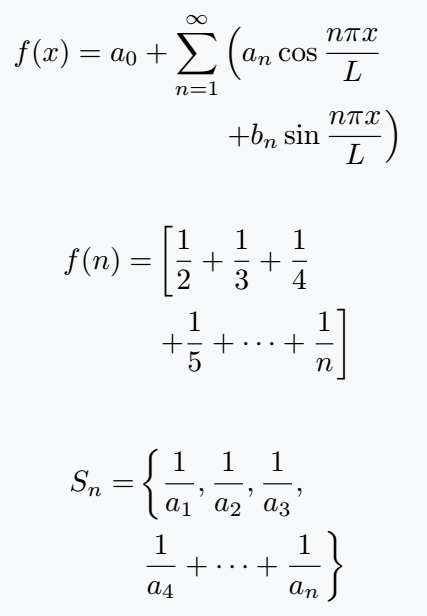You want to start in the second line from the bottom of any position on the first line. In both cases, you have to mark with & symbol.

## Use physics package for Big Brackets

I always think it is best practice to use a physics package in case of big brackets. Because you can complete the task by using a single command instead of writing multiple commands.

\documentclass{article}
\usepackage{physics}
\begin{document}
$\phi = a\cos \qty(\cos\qty(\frac{2\pi t+\phi}{A})A-2\pi ft)$
$\tan^{-1}\qty[\frac{2\qty[\frac{1-x}{1+x}]}{1-\qty[\frac{1-x}{1+x}]^2}]$
$\vb{Q} = \qty{\frac{a}{b}|a,b \; \epsilon \; \vb{Z}, b\neq 0}$
\end{document}

Output :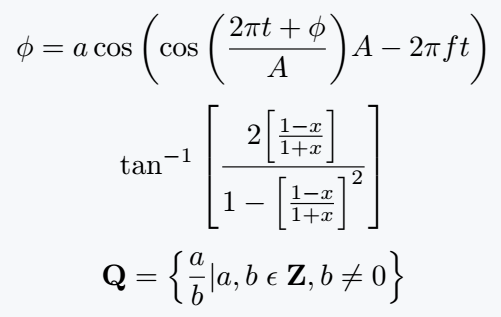Physics doesn’t need to be a big command, but it’s good to know.

\documentclass{article}
\usepackage{physics}
\begin{document}
$r = (I_n - (.85)M^T)^{-1}\qty\Big(\frac{1-(.85)}{5}).1$
$\int_1^\infty \frac{1}{x^2}dx = \qty\Big[-\frac{1}{x}]_1^\infty = 1$
${a_n} = \qty\Big{-\qty\Big(\frac{1}{n})^n}$
\end{document}

Output :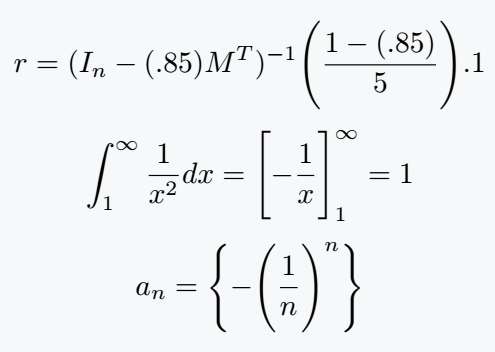If the size and shape of the expression are large, the size of the bracket should also be large. And big brackets will be needed in the latex document to represent multiple line expressions.

\documentclass{article}
\begin{document}
$(a), [b], \{c\}, \langle d \rangle$
$(\frac{X_i}{K_1})$
$\sum_{i=1}^n [\frac{x}{y_n}]$
$\{ \frac{1}{2}, \frac{1}{3}, \frac{1}{4}, \dots \}$
$\langle \frac{2}{3}, \frac{3}{4} \rangle$
\end{document}

Output :Notice the output above where your problem is described. And below is the use of \left and \right command to solve your problem.

\documentclass{article}
\begin{document}
$\left(\frac{X_i}{K_1}\right)$
$\sum_{i=1}^n \left[\frac{x}{y_n}\right]$
$\left\{ \frac{1}{2}, \frac{1}{3}, \frac{1}{4}, \dots \right\}$
$\left\langle \frac{2}{3}, \frac{3}{4} \right\rangle$
\end{document}

Output :For Big Brackets, there is no need to write large syntax in a latex document more than once. Instead, you can create small macros.

\documentclass{article}
\newcommand\Pbracket{\left(#1\right)}
\newcommand\Sbracket{\left[#1\right]}
\newcommand\Cbracket{\left\{#1\right\}}
\newcommand\Lbracket{\left\langle#1\right\rangle}
\begin{document}
$\Pbracket{\frac{X_i}{K_1}}$
$\sum_{i=1}^n \Sbracket{\frac{x}{y_n}}$
$\Cbracket{\frac{1}{2}, \frac{1}{3}, \frac{1}{4}, \dots}$
$\Lbracket{\frac{1}{2}, \frac{3}{4}}$
\end{document}

Output :## Various types of big commands in LaTeX

There are four types of big commands that do not depend on the expression. Size of bracket is not dynamically adjustable with the size of expression.

\documentclass{article}
\begin{document}
$\big(\frac{a_1}{k}\big), \Big(\frac{a_2}{k}\Big), \bigg(\frac{a_3}{k}\bigg), \Bigg(\frac{a_4}{k}\Bigg)$
$\big[\frac{b_1}{k}\big], \Big[\frac{b_2}{k}\Big], \bigg[\frac{b_3}{k}\bigg], \Bigg[\frac{b_4}{k}\Bigg]$
$\big\{\frac{c_1}{k}\big\}, \Big\{\frac{c_2}{k}\Big\}, \bigg\{\frac{c_3}{k}\bigg\}, \Bigg\{\frac{c_4}{k}\Bigg\}$
$\big\langle\frac{d_1}{k}\big\rangle, \Big\langle\frac{d_2}{k}\Big\rangle, \bigg\langle\frac{d_3}{k}\bigg\rangle, \Bigg\langle\frac{d_4}{k}\Bigg\rangle$
\end{document}

Output :## Use Big Brackets in multiple lines equation

No matter what environment you use, LaTeX has some rules for using big brackets with multiline equations.

\left and \right will be used simultaneously in each line. So, if there is no \right command at the end of a line and \left command at the beginning, you have to use \right. at the end of the line and \left. at the beginning.

\documentclass{article}
\usepackage{amsmath}
\begin{document}
\begin{align*}
f(x) = a_0 + \sum_{n=1}^\infty & \left( a_n\cos\frac{n\pi x}{L} \right. \\
&\left. + b_n\sin\frac{n\pi x}{L} \right)
\end{align*}
\begin{align*}
f(n) =  & \left[\frac{1}{2} + \frac{1}{3} +\frac{1}{4} \right. \\
&\left. + \frac{1}{5} + \cdots + \frac{1}{n} \right]
\end{align*}
\begin{align*}
S_n =  & \left\{\frac{1}{a_1}, \frac{1}{a_2}, \frac{1}{a_3}, \right. \\
&\left. \frac{1}{a_4} + \cdots + \frac{1}{a_n} \right\}
\end{align*}
\end{document}

Output :You want to start in the second line from the bottom of any position on the first line. In both cases, you have to mark with & symbol.

## Use physics package for Big Brackets

I always think it is best practice to use a physics package in case of big brackets. Because you can complete the task by using a single command instead of writing multiple commands.

\documentclass{article}
\usepackage{physics}
\begin{document}
$\phi = a\cos \qty(\cos\qty(\frac{2\pi t+\phi}{A})A-2\pi ft)$
$\tan^{-1}\qty[\frac{2\qty[\frac{1-x}{1+x}]}{1-\qty[\frac{1-x}{1+x}]^2}]$
$\vb{Q} = \qty{\frac{a}{b}|a,b \; \epsilon \; \vb{Z}, b\neq 0}$
\end{document}

Output :Physics doesn’t need to be a big command, but it’s good to know.

\documentclass{article}
\usepackage{physics}
\begin{document}
$r = (I_n - (.85)M^T)^{-1}\qty\Big(\frac{1-(.85)}{5}).1$
$\int_1^\infty \frac{1}{x^2}dx = \qty\Big[-\frac{1}{x}]_1^\infty = 1$
${a_n} = \qty\Big{-\qty\Big(\frac{1}{n})^n}$
\end{document}

Output :#### Md Jidan Mondal

LaTeX expert with over 10 years of experience in document preparation and typesetting. Specializes in creating professional documents, reports, and presentations using LaTeX.